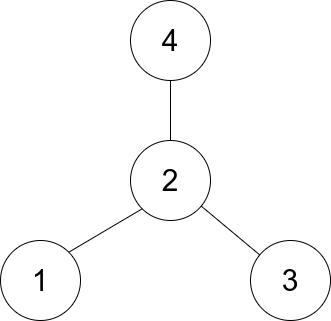1791. Find Center of Star Graph

# 1791.Find Center of Star Graph#

## 题目 #

There is an undirected star graph consisting of n nodes labeled from 1 to n. A star graph is a graph where there is one center node and exactly n - 1 edges that connect the center node with every other node.

You are given a 2D integer array edges where each edges[i] = [ui, vi] indicates that there is an edge between the nodes ui and vi. Return the center of the given star graph.

Example 1:``````Input: edges = [[1,2],[2,3],[4,2]]
Output: 2
Explanation: As shown in the figure above, node 2 is connected to every other node, so 2 is the center.
``````

Example 2:

``````Input: edges = [[1,2],[5,1],[1,3],[1,4]]
Output: 1
``````

Constraints:

• 3 <= n <= 100000
• edges.length == n - 1
• edges[i].length == 2
• 1 <= ui, vi <= n
• ui != vi
• The given edges represent a valid star graph.

## 解题思路 #

• 求出edges中前两个元素的共同值，即是中心节点

## 代码 #

``````package leetcode

func findCenter(edges [][]int) int {
if edges == edges || edges == edges {
return edges
}
return edges
}
``````Apr 8, 2023Edit this page